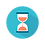From: Bayesian Models for Astrophysical Data, Cambridge Univ. Press

(c) 2017,  Joseph M. Hilbe, Rafael S. de Souza and Emille E. O. Ishida

you are kindly asked to include the complete citation if you used this material in a publication

Code 5.13  Beta model in R using JAGS

============================================================

require(R2jags)

# Data
set.seed(1056)                                                      # set seed to replicate example
nobs<-3000                                                          # number of obs in model
x1 <- runif(nobs)                                                  # random normal variable

# generate toy data
xb <- 0.3 + 1.5 * x1
exb <- exp(-xb)
p <- exb/(1+exb)
theta <- 15
y <- rbeta(nobs, theta*(1-p), theta*p)

# construct data dictionary
X <- model.matrix(~1 + x1)
K <- ncol(X)

model.data <- list(Y = y,                                     # response variable
X = X,                                   # predictor matrix
K = K,                                   # number of predictors including the intercept
N = nobs                               # sample size
)

Beta<-"model{
# Diffuse normal priors for predictors
for (i in 1:K) { beta[i] ~ dnorm(0, 0.0001) }

# Gamma prior for precision parameter
theta ~ dgamma(0.01,0.01)

# Likelihood function
for(i in 1:N){
Y[i] ~ dbeta(shape1[i],shape2[i])
shape1[i] <- theta*pi[i]
shape2[i] <- theta*(1-pi[i])
logit(pi[i]) <- eta[i]
eta[i] <- inprod(beta[],X[i,])
}
}"

# A function to generate initial values for mcmc
inits <- function () { list(beta = rnorm(ncol(X), 0, 0.1)) }

# Parameters to be displayed in output
params <- c("beta""theta")

BETAfit<- jags(data = model.data ,
inits = inits,
parameters = params,
model = textConnection(Beta),
n.thin = 1,
n.chains = 3,
n.burnin = 2500,
n.iter = 5000)

print(BETAfit, justify="left", digits=2)

============================================================Output on screen:

Inference for Bugs model at "4", fit using jags,

3 chains, each with 5000 iterations (first 2500 discarded)

n.sims = 7500 iterations saved

mu.vect     sd.vect          2.5%            25%             50%             75%          97.5%     Rhat       n.eff

beta                0.30       0.02             0.27             0.29              0.30             0.32             0.34           1      1500

beta                1.48       0.03             1.41             1.45              1.48             1.50             1.55           1      1400

theta                  15.40       0.40           14.62           15.13            15.39           15.66           16.17          1      7500

deviance      -5173.77       2.43      -5176.56      -5175.56      -5174.36      -5172.67      -5167.56          1       700

For each parameter, n.eff is a crude measure of effective sample size,

and Rhat is the potential scale reduction factor (at convergence, Rhat=1).

DIC info (using the rule, pD = var(deviance)/2)

pD = 2.9 and DIC = -5170.8

DIC is an estimate of expected predictive error (lower deviance is better).